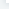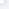# R语言中plot函数的使用

：2018-07-07 11:05:52

R有强大的绘图功能，R提供的绘图函数可以分为三种基本类型：高水平绘图函数、低水平绘图函数和交互式绘图函数。

R语言中plot()函数的基本格式如下：

plot(x,y,...)

plot函数中，x和y分别表示所绘图形的横坐标和纵坐标；

plot函数默认的使用格式如下：

plot(x, y = NULL, type = "p", xlim = NULL, ylim = NULL,
log = "", main = NULL, sub = NULL, xlab = NULL, ylab = NULL,
ann = par("ann"), axes = TRUE, frame.plot = axes,
panel.first = NULL, panel.last = NULL, asp = NA, ...)

（1）type为一个字符的字符串，用于给定绘图的类型，可选的值如下：

"p"：绘点（默认值）；

"l"：绘制线；

"b"：同时绘制点和线；

"c"：仅绘制参数"b"所示的线；

"o"：同时绘制点和线，且线穿过点；

"h"：绘制出点到横坐标轴的垂直线；

"s"：绘制出阶梯图（先横后纵）；

"S"：绘制出阶梯图（先纵后竖）；

"n"：作空图。

（2）main参数

（3）sub参数

（4）xlab参数

（5）ylab参数

（6）xlim参数

（7）ylim参数

（1）绘制简单的一个点

plot(10,10) #直接给出数据

#使用变量的情况
x<-10
y<-10
plot(x,y)

（2）绘制一条线

x<-c(20,30)
y<-c(40,50)
plot(x,y)

（3）使用参数l——绘制一条直线

plot(x,y,'l')

（4）使用参数b——同时绘制点和线，线不穿过点

plot(x, y, 'b')

（5）使用参数c——仅绘制出参数为b时所示的直线

plot(x, y, "c")

（6）使用参数o

plot(x, y, "o")

（7）使用参数h

plot(x, y, "h")

（8）使用参数s——阶梯图，先横再纵

plot(x, y, "s")

（9）使用参数S

plot(x, y, "S")

（10）使用main参数、xlab,ylab的情形

plot(x, y, "l",main="plot函数绘图演示",xlab="x值",ylab="y值")

（11）xlim、ylim参数的使用

plot(x,y,xlim=c(10,50),ylim=c(10,50))

 编号 1 2 3 4 5 6 7 8 9 10 消费支出 20 15 40 30 42 60 65 70 53 78 可支配收入 25 18 60 45 62 88 92 99 75 98

`x<-c(25,18,60,45,62,88,92,99,75,98) #定义向量x保存可支配收入y<-c(20,15,40,30,42,60,65,70,53,78) #定义向量y保存消费支出#绘制散点图plot(x,y,xlab="可支配收入",ylab="消费支出",main="消费支出与可支配收入的散点图")`

R语言中plot函数的使用

## 相关文章